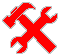#SysTools

## PHP: tiny helper dump log function

```<?php

/*
tiny helper dump log function - dumps values to the log file
helps a lot when debugging and understanding large projects
it's a good idea to place it in some PHP file which always included
as example - this code may be put in some config.php / init.php / etc.

// few examples of usage

// just dump something
dumptemp(time());
// dump to use it later
dumptemp(\$_POST, true);
// check where the code execution goes
if (intval(date('H')) <= 12) {
dumptemp('goes to AM branch');
// some code here
} else {
dumptemp('goes to PM branch');
// some code here
}
// check that this place can be reached
dumptemp('AM/PM branch done');

*/

function dumptemp(\$value, \$usable = false) {
if (defined('__DUMPTEMP__')) {
\$mode = 'a';
} else {
// log file will be recreated for the each script run
\$mode = 'w';
define('__DUMPTEMP__', true);
}
// replace log file name and path here
\$fl = @fopen('/tmp/dumptemp', \$mode);
if (\$fl) {
// if usable not false - serialize value so it's contents can be easily used later
fwrite(\$fl, ((\$usable !== false) ? serialize(\$value) : print_r(\$value, true)).PHP_EOL);
fclose(\$fl);
}
}
```

2016.08.05

[ Код ]